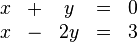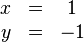# Linear algebra facts for kids

Kids Encyclopedia Facts

Linear algebra is a branch of mathematics. It came from mathematicians trying to solve systems of linear equations. Vectors and matrices are used to solve these systems. The main objects of study currently are vector spaces and linear mappings between vector spaces. Linear algebra is useful in other branches of mathematics (e.g. differential equations and analytic geometry). It can also be applied to the real world in areas such as engineering, physics and economics.

Linear algebra describes ways to solve and manipulate (rearrange) systems of linear equations.

For example, consider the following equations:$\begin{matrix} x &+& y &=& 0 \\ x &-& 2y &=& 3 \end{matrix}$

These two equations form a system of linear equations. It is linear because none of the variables are raised to a power. The graph of a linear equation in two variables is a straight line. The solution to this system is:$\begin{matrix} x &=& 1 \\ y &=& -1 \end{matrix}$

since it makes all of the original equations valid, that is, the value on left side of the equals sign is exactly the same as the value on the right side for both equations.

Linear algebra uses a system of notation for describing system behavior, called a matrix. For the previous example, the coefficients of the equations can be stored in a coefficient matrix.Linear algebra Facts for Kids. Kiddle Encyclopedia.